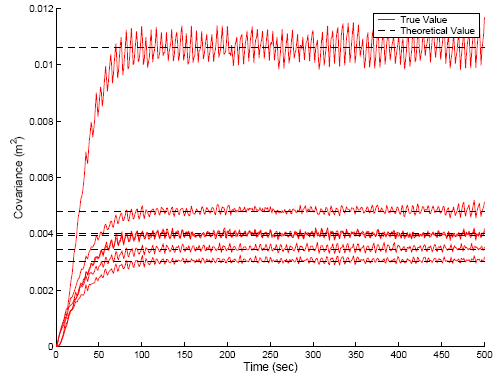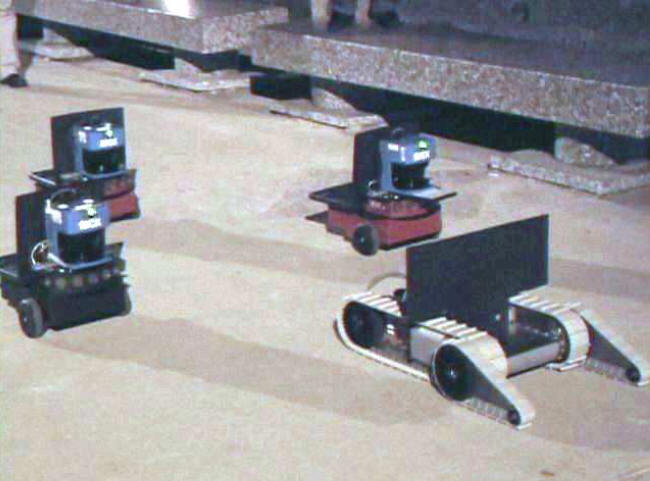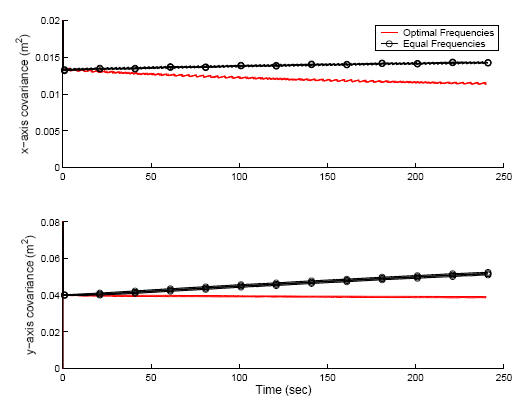## Optimal Sensing Strategies for Mobile Robot Formations

• Motivation

• This work addresses the problem of Cooperative Localization (CL) in robot formations. In particular, our goal is to determine optimal sensing strategies for the robot team, that maximize the estimation accuracy under constraints imposed by the limited computational and communication resources.
We examine the case where data fusion is carried out by a centralized extended Kalman filter (EKF) estimator, which optimally combines the odometry and exteroceptive (e.g., GPS, compass, relative-position) measurements of all robots. In this scenario, each robot's odometry measurements can be processed locally by the robot, to propagate its own pose estimates. However, every time an exteroceptive measurement is received by any of the robots in the formation, all robots must communicate their current pose estimates. Additionally, the measuring robot must transmit its new measurement in order for an EKF update to be carried out.
Therefore, every exteroceptive measurement that is processed incurs a penalty in terms of use of communication bandwidth, CPU time, and power consumption. In a realistic scenario, the robots of a team will need to allocate computational and communication resources to mission-specific tasks, and this may force them to reduce the number of measurements they process for localization purposes. Moreover, the finite battery life of robots imposes constraints on the amount of power that can be used for tracking their position. The limitations on the available resources may thus prohibit the robots from registering, transmitting, and processing all measurements available at every time instant. Clearly, a framework for optimizing the estimation accuracy under the given constraints is required.
• Approach

• Whether or not an exteroceptive measurement should be processed in an EKF update, is determined by a tradeoff between the value of the localization information it carries, and the cost of processing it. In this paper, we assume that the robots process each of the available measurements at a constant frequency. Our goal is to determine the optimal frequency for each available measurement, so as to attain the highest possible positioning accuracy.
Our analysis is based on examining the "rate of information" that each particular measurement contributes to the estimator. Based on this analysis, we can derive an equivalent, continuous-time system model for the robot team, whose noise parameters are functionally related to the frequency of the measurements. This is demonstrated in the following figure:In this figure, the red lines show the time evolution of the diagonal elements of the covariance matrix during CL. Due to the fact that different measurements are processed at every time step, the elements of the covariance matrix fluctuate. However, that after some time a steady state is reached, where the covariance matrix elements fluctuate around constant values (for an observable system). The dashed black lines in the figure show the steady-state covariance values computed using the equivalent continuous-time system. It is clear that the continuous-time analysis gives an excellent description of the mean steady-state covariance in the actual, time-varying system.
• Contribution
• The novel formulation proposed in our work enables us to express the covariance matrix of the pose errors as a functional relation of the frequencies, and thus to formulate the problem of determining the optimal sensing strategy as an optimization one. An important result that we prove is that this is a convex optimization problem (SDP) and therefore it is possible to compute a globally optimal solution, using very efficient algorithms.
Our approach makes it possible to determine the optimal sensing strategy that satisfies any application-imposed constraints on communication, power, and processing resources. In addition, the results of this work may also be employed to reduce the cost of a robot team design. Specifically, if measurements from certain active sensors (e.g., lasers) are processed at a low rate, it may be possible to replace these particular sensors with slower (and cheaper) ones. Finally, in the event that the utilization frequency of a specific sensor is determined, through the optimization process, to be approximately zero, this sensor may be omitted altogether, thus reducing the cost of the robots' sensing payload.
• Results

•To validate the theoretical analysis, we have conducted several experiments in simulation, as well as real-world experiments with a heterogeneous robot team, comprised of one iRobot Packbot robot and 3 Pioneer-I robots. The robots move outdoors in a diamond-shaped formation, where the Packbot is the “leader”, as shown above. Each of the Pioneers is equipped with a laser scanner, and is able to detect the robots of the team that lie within its field of view. Using a linefitting technique, we are able to extract relative position (i.e., range and bearing) as well as relative orientation information. In addition to the relative-pose measurements, absolute position and orientation measurements are provided to the team by a GPS receiver and a magnetic compass, which are mounted on the Packbot.
Our method has been applied to determine the optimal sensing frequencies for the robot team, and the accuracy of the derived sensing strategy was compared against the accuracy of an "intuitively good" strategy, where all available sensors are used at equal rates. The comparison of performance is shown below:In this plot, the variance of the position errors along the x- and y- axes for all robots is shown, as a function of time, The red lines correspond to the optimal frequencies obtained by our method, while the black ones correspond to the "intuitive strategy" of equal frequencies. As evident, there is a clear improvement of performance by using the frequency values produced by the proposed algorithm. Evaluating the steady-state covariance attained with the equal-frequency strategy shows that it is approximately 130% and 50% larger along the x and y axes, respectively, compared to the optimal values obtained with our approach.
• Relevant Publications
• C2. A.I. Mourikis, S.I. Roumeliotis: "Optimal Sensor Scheduling for Resource-Constrained Localization of Mobile Robot Formations,''  IEEE Transactions on Robotics 22(5), pp. 917-931, Oct. 2006.
• C1. A.I. Mourikis, S.I. Roumeliotis: "Optimal Sensing Strategies for Mobile Robot Formations: Resource-Constrained Localization," Proceedings of Robotics: Science and Systems, June 8-11, 2005, Boston, MA, pp. 281-288.
• Acknowledgements
• This work was supported by the University of Minnesota (DTC), the Jet Propulsion Laboratory (Grant No. 1251073, 1260245, 1263201), and the National Science Foundation (ITR-0324864, MRI-0420836).